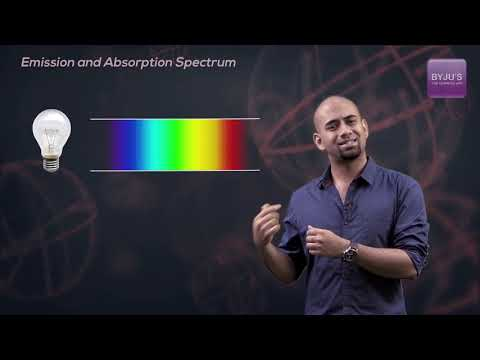Jet Set Go! All about Aeroplanes Jet Set Go! All about Aeroplanes

# Atomic Spectra Questions

We know that electrons have discrete and specific energies in an atom. An atom has more energy states than electrons. When an electron transitions from one energy level to another, it emits light or photons of a specific wavelength. The atomic spectrum is the collection of all these specific wavelengths under any given set of conditions. As a result, atomic spectra are atom spectra.

Definition: Atomic spectra is defined as the electromagnetic radiation spectrum emitted or absorbed by an electron as it transitions between different energy levels within an atom.

## Atomic Spectra Chemistry Questions with Solutions

Q-1: Atomic spectra is also known as

a) continuous spectra

b) line spectra

c) Spectra of absorption

d) The emission spectrum

Explanation: Line spectra are obtained as a result of absorption and subsequent emission of energy by the electrons in the individual atoms of the element. Hence, the line spectrum is also called atomic spectrum.

Q-2: The atomic spectrum of Li2+ should be similar to that of

a) He+

b) Na

c) He

d) H

Explanation: The atomic spectrums of mono electronic species are identical. A mono electronic species is a specie that contains only one electron.

He+ and H contain only one electron. Hence their atomic spectrum is similar to mono electronic specie Li2+.

Q-3: Calculate the radius ratio for 2nd orbit of He+ ion and 3rd orbit of Be+++ ion.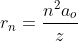For He+ ion, z=2, n=2( 2nd orbit)

For Be+++ ion, z=4, n= 3 (3rd orbit)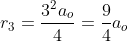The radius ratio for 2nd orbit of He+ ion and 3rd orbit of Be+++ ion comes out to be 8/9.

Q-4: The energy of an electron in a hydrogenic atom with nuclear charge Z varies as

a) Z

b) Z2

c) 1/Z

d) 1/Z2

Explanation: The expression for the energy calculation of an electron in a hydrogenic atom with nuclear charge Z and orbit number, n is given by: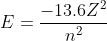As a result, we can clearly see that it is proportional to Z2.

Q-5:The potassium salts are placed in a Bunsen flame to obtain the line spectrum, the colour of the light emitted is

a) yellow

b) violet

c) green

d) white

Explanation: The colour of the light emitted depends upon the nature of the substance. For example, sodium and its salts emit yellow light while potassium or its salts give out violet light.

Q-6: Name the gas that is used in the discharge tube to obtain the hydrogen absorption spectrum?

Explanation: When H2 gas is introduced into the discharge tube, a series of lines such as lyman, balmer, paschen, bracket, and pfund that are located in different regions are obtained.

Q-7: A 𝛼- line of Balmer series corresponds to

a) shortest wavelength with n2=2

b) longest wavelength with n2=2

c) longest wavelength with n2=3

d) shortest wavelength with n2=3

Answer: c) longest wavelength with n2=3

Explanation: 𝛼- line of Balmer series is the first line of Balmer series.

For the Balmer series, n1 = 2 and for the first line, n2= n1+1 = 2+1 = 3

Q-8: Distinguish between the emission and absorption spectra.

Emission Spectra Absorption spectra
1) Emission spectrum is obtained when the radiation from the source is directly analysed in the spectroscope. 1) It is obtained when the white light is first passed through the substance and the transmitted light is analysed in the spectroscope.
2) It consists of bright coloured lines separated by dark spaces 2) It consists of dark lines.
3) It can be continuous or discontinuous. 3) It is always discontinuous.

Q-9: Calculate the wavelength of a photon emitted when an electron in H-atom makes a transition from n=2 to n=1.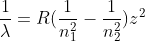Here, n1= 1 and n2=2 and R is the rydberg constant. Z is the atomic number of H-atom

Z=1 , R= 109677 cm-1

Substituting in the formula, we get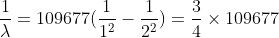Thus, 𝞴= 2.27 × 10-6 cm

Q-10: If an electron makes transition from the 7th excited state to 2nd state in a H-atom sample, find the maximum number of spectral lines observed.

Answer: The maximum number of spectral lines(N) can be calculated using the below formula: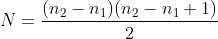Here, n2 = 8(for 7th excited state) and n1= 2. Substituting in the formula, we get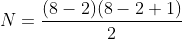N= 21

Q-11: The wavelength of lyman series lies in the

a) Visible region

c) Ultraviolet region

d) Infrared region

Q-12: What is the name of the equipment used to analyse sunlight by splitting it into its various colours?

a) monochromator

b) spitter

c) spectrometer

d) spectrograph

Explanation: A spectrograph, also known as a spectroscope or spectrometer, divides light from a single material into its component colours in the same way that a prism divides white light into a rainbow. It captures this spectrum, allowing scientists to analyse the light and learn about the properties of the material interacting with it.

Q-13: A line at 3802 cm-1 is obtained in the infrared region of the atomic spectrum of hydrogen. Determine the energy of this photon.

Answer: Given,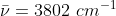𝝀 = (1/3802) cm

We know that, Energy, E= h𝝂= hc/𝝀

Where h is the planck’s constant and c is the speed of light,

h= 6.63×10-34Js , c= 3×108m/s = 3×1010cm/s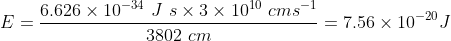Q-14: Which of the following is an example of line absorption spectrum?

a) Mercury vapour lamp

b) Sunlight Spectrum

c) Spectrum of oil flame

d) Solar spectrum

Answer: b) Sunlight Spectrum is an example of line absorption spectrum

Q-15: The correct order of Bohr radius is

a) r1>r2>r3>r4

b) r1<r2<r3<r4

c) r1=r2=r3=r4

d) r1<r2>r3<r4

Explanation: Bohr radius is represented by rn where n is the orbit number. It is given by the formula:As a result, as the value of n increases, so does the bohr radius.

Therefore the correct order is r1<r2<r3<r4

## Practise Questions on Atomic Spectra

Q-1: Which theory was able to explain the line spectra of hydrogen?

a) Dalton’s Atomic theory

b) Bohr’s theory

c) Rutherford’s model

d) Thomson’s Atomic model

Q-2: Which electronic level allows the hydrogen atom to absorb but not emit photons?

a) 3s

b) 2p

c) 2s

d) 1s

Q-3: Calculate wavelength for the 2nd line of the Balmer series of He+ ion.

Q-4: The principal quantum number of an atom is related to the

a) size of the orbital

b) orientation of the orbital in space

c) spin angular momentum

d) orbital angular momentum

Q-5: Calculate the frequency of light emitted when an electron drops from the higher to the lower state if the energy difference between the two electronic states is 214.68 kJ/mol. (Planck’s constant,h= 39.79× 10-14 kJs/mol)

Click the PDF to check the answers for Practice Questions.

## Structure of Atom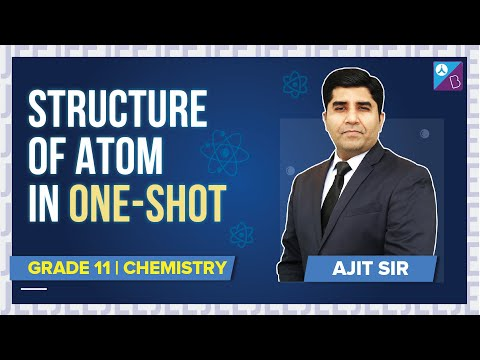## Emission and Absorption Spectrum